Question

# Complete and balance the molecular equation for the mixing of the following solutions: H2CrO4 (aq) +...

Complete and balance the molecular equation for the mixing of the following solutions:

H2CrO4 (aq) + Fe(HCO3)3 (aq) -->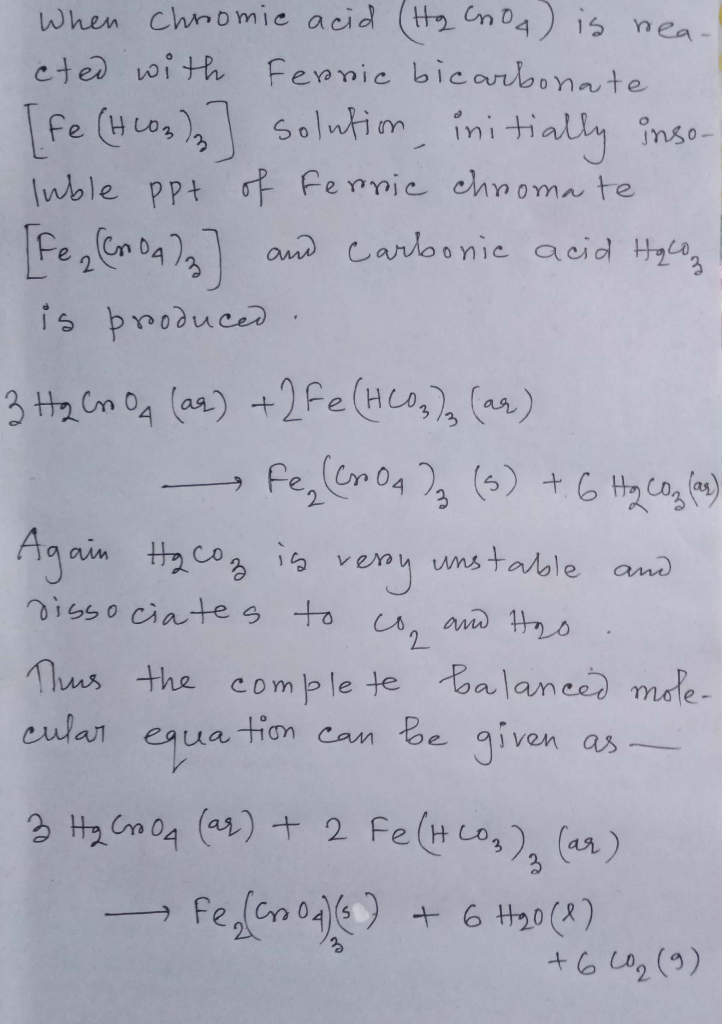#### Earn Coins

Coins can be redeemed for fabulous gifts.

Similar Homework Help Questions
• ### Complete and balance the following molecular equation. HBr(aq)+Ca(OH)2(aq)→ Express your answer as a balanced chemical equation....

Complete and balance the following molecular equation. HBr(aq)+Ca(OH)2(aq)→ Express your answer as a balanced chemical equation. Identify all of the phases in your answer. and write the ionic equation for it

• ### 11. NaC H30 (aq) and H.SO.(aq) Observations after mixing Balanced Molecular equation: 12. NH.Cl(aq) and NaOH(aq)...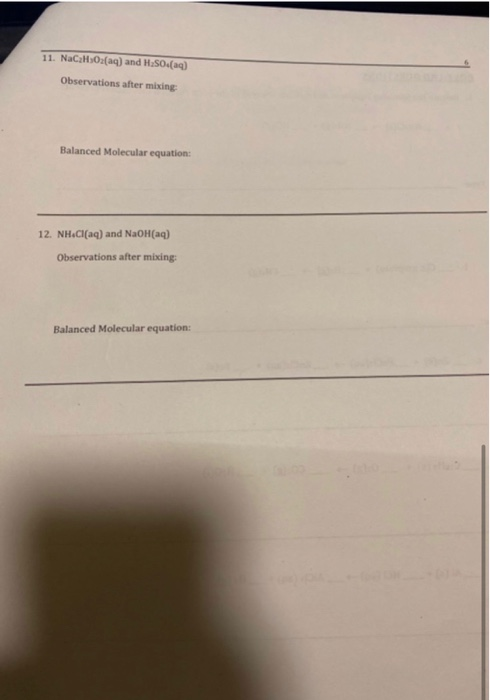11. NaC H30 (aq) and H.SO.(aq) Observations after mixing Balanced Molecular equation: 12. NH.Cl(aq) and NaOH(aq) Observations after mixing: Balanced Molecular equation:

• ### 1) first, write the complete molecular equation between the two aqueous solutions and balance the reaction....

1) first, write the complete molecular equation between the two aqueous solutions and balance the reaction. Then write the complete ionic equation and net ionic equation for this reaction.

• ### Complete the following molecular equation Pb(NO3)2(aq) + 2KI(aq) →

Complete the following molecular equation Pb(NO3)2(aq) + 2KI(aq) →

• ### Balance the following equation (and show your work), in basic solution:           ClO4-(aq) + C6H14O5(aq) →...Balance the following equation (and show your work), in basic solution:           ClO4-(aq) + C6H14O5(aq) → ClO-(aq) + HCO3-(aq) Balance the following equation (and show your work), in basic solution: CIO4 (aq) + CH120(aq) - CIO (aq) + HCO3(aq)

• ### The following molecular equation represents the reaction that occurs when aqueous solutions of silver(I) nitrate and...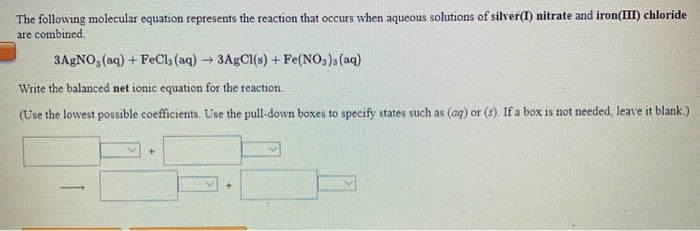The following molecular equation represents the reaction that occurs when aqueous solutions of silver(I) nitrate and iron(III) chloride are combined 3AgNO3(aq) + FeCl3(aq) → 3AgCl(s) + Fe(NO3), (aq) Write the balanced net ionic equation for the reaction. (Use the lowest possible coefficients. Use the pull down boxes to specify states such as (aq) or (\$). If a box is not needed, leave it blank.)

• ### 1. Balance the molecular equation below and write the balanced complete and net ionic equations for...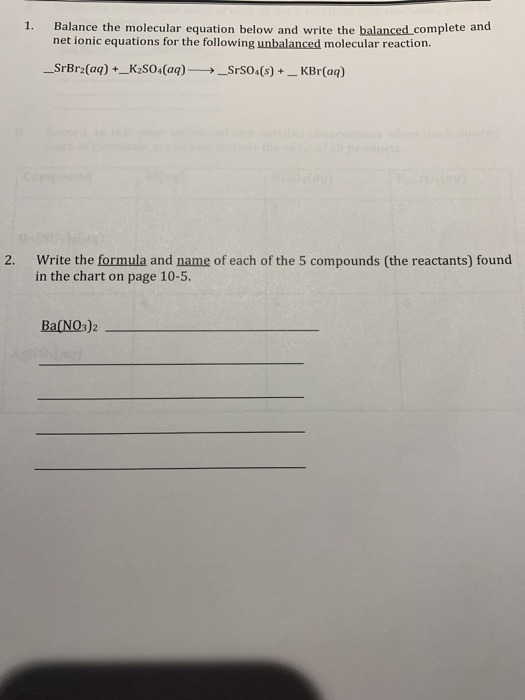1. Balance the molecular equation below and write the balanced complete and net ionic equations for the following unbalanced molecular reaction. _SrBr2(aq) +_K2SO4(aq) → _SrS04(8) + _ KBr(aq) 2. Write the formula and name of each of the 5 compounds (the reactants) found in the chart on page 10-5. Ba(NO3)2 Name TA Partner Date 10 Data Sheets A Write the formula and color of each of the solutions the reactants) given in the chart below. Ba(NO) colorless B. Record, in...

• ### Complete and balance the molecular equation for the reaction of aqueous sodium carbonate and aqueous nickel Chloride In...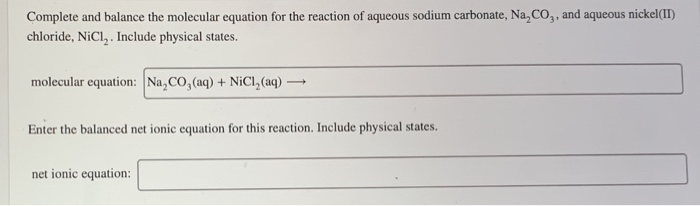Complete and balance the molecular equation for the reaction of aqueous sodium carbonate and aqueous nickel Chloride Include physical states Complete and balance the molecular equation for the reaction of aqueous sodium carbonate, Na, Co,, and aqueous nickel(II) chloride, NiCl. Include physical states. molecular equation: Na, CO, (aq) + NiCI, (aq) + Enter the balanced net ionic equation for this reaction. Include physical states. net ionic equation:

• ### Balance the following equation (and show your work), in basic solution: ClO4-(aq) + C6H14O5(aq) → ClO-(aq)...

Balance the following equation (and show your work), in basic solution: ClO4-(aq) + C6H14O5(aq) → ClO-(aq) + HCO3-(aq)

• ### Balance the following equation (and show your work), in basic solution:             ClO4-(aq) + C6H14O5(aq) →...

Balance the following equation (and show your work), in basic solution:             ClO4-(aq) + C6H14O5(aq) → ClO-(aq) + HCO3-(aq)